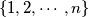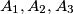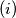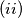### IMO Shortlist 1987 problem 11

Kvaliteta:
Avg: 0,0
Težina:
Avg: 0,0
Find the number of partitions of the set$\{1, 2, \cdots, n\}$ into three subsets$A_1,A_2,A_3$, some of which may be empty, such that the following conditions are satisfied:$(i)$ After the elements of every subset have been put in ascending order, every two consecutive elements of any subset have different parity.$(ii)$ If$A_1,A_2,A_3$ are all nonempty, then in exactly one of them the minimal number is even .

Proposed by Poland.
Izvor: Međunarodna matematička olimpijada, shortlist 1987